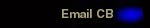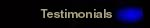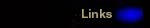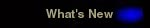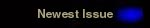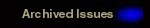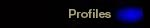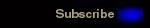ISSUE #2

INSIDE THIS ISSUE...

1 - STRENGTH TESTING TECHNIQUES & ESTIMATIONS

The beginning of the training year of off-season are excellent times to determine your strength levels. Re-testing later in the year can help to determine the effectiveness of your program.

This estimation formula works extremely well with the bench press. Choose a weight you can bench for 6-8 reps then enter it as the "sub-maximal weight".

1 RM calculation

Sub-maximal weight x .03 = SW1

SW1 x # of reps completed = SW2

SW2 + Sub-maximal weight = theoretical maximum (1 RM)

For example:

185 lbs. x 6 repetitions

185 x .03 = 5.55

5.55 x 6 = 33.3

33.3 + 185 = 218 lbs.

Therefore the predicted maximum bench press is 218 lbs.

2 - AEROBIC FITNESS TESTING & CALORIC EXPENDITURE ESTIMATIONS

Aerobic fitness is measured as VO2max (maximal oxygen uptake) and this attribute can be estimated from your performance in a 12- to 15-minute run. You must know the exact time and distance of the run (converted to meters).

Determine the average velocity of the run (for example a 15-min run):

Average velocity = 15 min run distance (meters)/15 min

= meters per minute

ml = milliliters       kg = kilograms      min = minutes

Use the average velocity in the following equation to estimate the VO2:

VO2max (ml/kg/min) = 33.3 + (average velocity - 150) x 0.178 ml/kg/min

You now have an estimation of your aerobic fitness in relation to your bodyweight. The average VO2 of an active college male is ~ 40-50 ml/kg/min and is slightly lower for active females.

To determine the number of calories you burned in an exercise session you must first convert your aerobic fitness estimation to L/kg/min (so divide your score by 1000). For example:      50 ml/kg/min  --> 0.050 L/kg/min

Then insert the appropriate values (body weight and exercise duration) in the following equation. For VO2, you need to estimate a percent intensity at which you were working. For example, on a hard run you might say you were exercising at 80% VO2max whereas after a brisk walk you would likely be exercising at only 50% VO2max.

Calories burned = VO2 (L/kg/min) x wt (kg) x time (minutes) x 5 (kcal/min/LO2)

5 (kcal/min/LO2) = the number of calories used per liter of oxygen consumed.

So for example, a 70 kg subject with a 50 ml/kg/min VO2max exercising at 75% VO2max for 30 minutes:

Calories Burned = (0.050 x .75) x 70 x 30 x 5

= 0.0375 x 70 x 30 x 5

= 394 kcal

3 - ECCENTRIC CONTRACTIONS: POSITIVE RESULTS FROM "NEGATIVES"

An eccentric contraction is defined as the lowering of the weight and therefore considered a "negative". Everyone is stronger in this action in comparison to the concentric (lifting) action and eccentric training results in greater muscle damage and is responsible for the muscle soreness felt in the following days after a hard training session. The benefits of eccentric training may be a greater stimulus for strength and muscle growth development.

To perform "negatives", choose a weight that you have the ability to lower for 8 seconds. If it can't be lowered for more than 3 seconds, the weight is too heavy, the set should be ended, and a lower weight used for the next set.

Eccentrics can cause great damage and soreness and should be used only in low volume. Begin by adding 3-4 eccentric repetitions to a normal set and progress to a maximum of 2 eccentric sets per workout. As well, eccentrics should only be trained for 1-2 body parts simultaneously (1-2 muscle groups per week). Allow the muscles to fully recover before training them again in this manner.

CB Athletic Consulting, Inc.